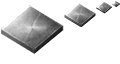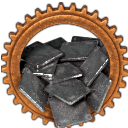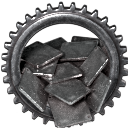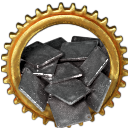铁矿 ×1

3.2

MOD开发

## 相关成就#### 钢铁大亨 1#### 钢铁大亨 2×1
×1
×1
×1
0.5
×2
×1
×1
0.5
×1
×1
×1
0.5
×1
×1
×3
×1
0.5
×1
×1
×1
×1
0.5
×1
×1
×3
0.5
×1
×1
0.5
×2
×1
0.5
×50
×1
×5
×100
1
×1
×1
×1
×20
4
×1
×2
0.5
×1
×2
×2
×1
0.5
×1
×2
×2
3
×1
×2
×1
×1
8
×1
×2
×5
10
×1
×3
×3
×1
2
×1
×3
×5
×4
×4
2
×1
×4
1
×2
×5
×10
0.5
×1
×5
×1
0.5
×1
×5
×1
0.5
×1
×5
×5
×4
1
×1
×5
×5
×2
2
×1
×5
×5
5
×1
×5
×10
8
×1
×5
×5
×5
10
×1
×5
16
×1
×6
×3
×6
×5
0.5
×1
×8
0.5
×1
×9
×5
×3
0.5
×1
×10
×8
×5
0.5
×1
×10
×5
×5
0.5
×2
×10
×5
1
×1
×10
×5
×3
2
×1
×10
×5
×10
10
×10
×10
×1
×19
10
×1
×15
×5
×10
×5
10
×1
×20
×20
×10
1
×1
×20
×5
×8
2
×1
×20
×5
3
×1
×20
×10
×10
8
×1
×40
3
0.0
0人评价